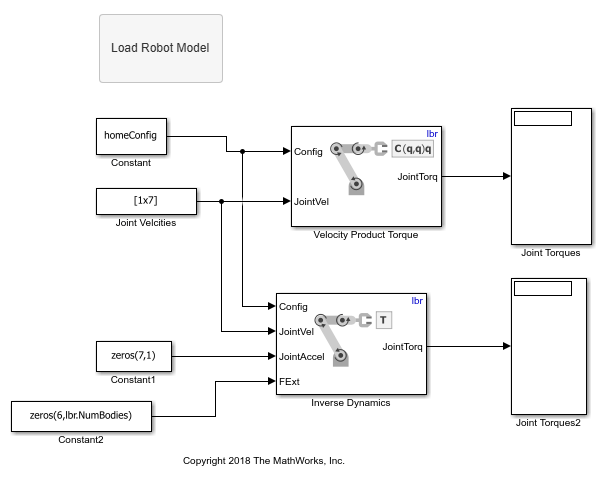Compute Velocity Product for Manipulators in Simulink

This example shows how to calculate the velocity-induced torques for a robot manipulator by using a rigidBodyTree model. In this example, you define a robot model and robot configuration in MATLAB® and pass them to Simulink® to be used with the manipulator algorithm blocks.

Load a RigidBodyTree object that models a KUKA LBR robot. Use the homeConfiguration function to get the home configuration or home joint positions of the robot.

lbr.DataFormat = 'column';

homeConfig = homeConfiguration(lbr);

Open the model. If necessary, use the Load Robot Model callback button to reload the robot model and configuration vector.

open_system('velocity_product_example.slx')Run the model. The Velocity Product block calculates the torques induced by the given velocities. Verify these values by passing the same velocities to the Inverse Dynamics block with no acceleration or external forces.